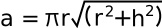#Surface Area of a Cone Calculator

 Radius: units Height: units Area: units2

This application calculates the surface area of a cone, not including the base. This is sometimes called the "lateral surface of the cone". The formula for calculating the surface area of a cone is:where:
r = radius of the base of the cone
h = height of the cone
a = area of surface

To calculate the entire surface of the cone, add the area of the base, which is simply the area of a circle. When entering numbers, do not enter the units. The units can be considered any units, as long as they are all the same unit. Do not enter commas in your numbers.